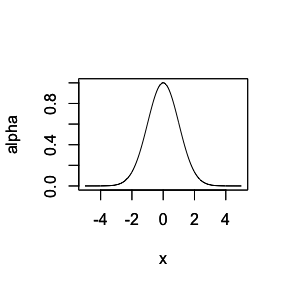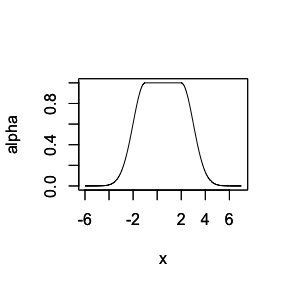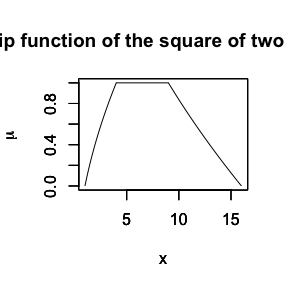# On fuzzification tools, fuzzy arithmetics and metrics

library(FuzzySTs)
#> Loading required package: FuzzyNumbers
#> Loading required package: polynom
#>
#> Attaching package: 'FuzzySTs'
#> The following object is masked from 'package:FuzzyNumbers':
#>
#>     distance

## is.alphacuts(): Verifies if a matrix is set of left and right alpha-cuts

FuzzySTs::is.alphacut() verifies if a given matrix can be a matrix of numerical alpha-cuts. It is then verified if the considered matrix has the following requirements: it should be composed by 2 vectors in columns with no missing values, for which the lengthes of both vectors are equal. The first vector should be increasing and named “L” as left, and the second one should be decreasing and named “U” as upper. The last value of the first vector should be less than the first value of the second vector.

# Simple example
mat <- matrix(c(1,2,3,7,6,5), ncol = 2)
is.alphacuts(mat)
#>  TRUE

## nbreakpoints(): Calculates the number of breakpoints of a numerical matrix of alpha-cuts

For a given fuzzy number expressed by its numerical alpha-cuts such that is.alphacuts = TRUE, the function FuzzySTs::nbreakpoints() calculates the number of breakpoints chosen to numerically construct these alpha-cuts. In other terms, it is the number of sample points on which the membership functions are evaluated.

# Simple example
X <- TrapezoidalFuzzyNumber(1,2,3,4)
alpha.X <- alphacut(X, seq(0,1,0.01))
nbreakpoints(alpha.X)
#>  100

## GaussianFuzzyNumber(): Creates a Gaussian fuzzy number

FuzzySTs::GaussianFuzzyNumber() creates a Gaussian fuzzy number from the class of Gaussian fuzzy numbers. This number is given by its numerical alpha-cuts such that is.alphacuts = TRUE. The smoothness of the constructed curve are defined by the step, the margin and the precision fixed by default to 0.01, [3 * sigma; 3 * sigma] and 4.

# Simple example
GFN <- GaussianFuzzyNumber(mean = 0, sigma = 1, alphacuts = TRUE, plot=TRUE)is.alphacuts(GFN)
#>  TRUE

## GaussianBellFuzzyNumber(): Creates a Gaussian two-sided bell fuzzy number

FuzzySTs::GaussianBellFuzzyNumber() creates a two-sided Gaussian Bell fuzzy number from the class of Gaussian Bell fuzzy numbers. The constructed curve is a combination of two Gaussian curves. The smoothness of the constructed curve are defined by the step, the margin and the precision fixed by default to 0.01, [3 * left.sigma; 3 * right.sigma] and 4.

# Simple example
GBFN <- GaussianBellFuzzyNumber(left.mean = -1, left.sigma = 1, right.mean = 2, right.sigma = 1, alphacuts = TRUE, plot=TRUE)is.alphacuts(GBFN)
#>  TRUE

## Fuzzy.Difference(): Calculates the difference between two fuzzy numbers

FuzzySTs::Fuzzy.Difference() calculates the difference between two trapezoidal fuzzy numbers with respect to the extension principle. In case the fuzzy numbers are not trapezoidal, and written by their numerical alpha-cuts, the function constructs a trapezoidal approximation composed by the minimum and maximum values of the left and the right alpha-cuts of the fuzzy number.

# Simple example
X <- TrapezoidalFuzzyNumber(5,6,7,8)
Y <- TrapezoidalFuzzyNumber(1,2,3,4)
Fuzzy.Difference(X,Y)
#> Trapezoidal fuzzy number with:
#>    support=[2,6],
#>       core=[3,5].

## Fuzzy.Square(): Calculates numerically the square of a fuzzy number

FuzzySTs::Fuzzy.Square() calculates numerically the square of a fuzzy number with respect to the extension principle. The considered input fuzzy number is supposed to be trapezoidal or triangular. However, if a fuzzy number of some other types is introduced by its numerical alpha-cuts, a trapezoidal approximative fuzzy number is constructed by considering the minimum and maximum values of the left and the right alpha-cuts of the fuzzy number. The calculations are based on resolving second order polynoms.

# Simple example
X <- TrapezoidalFuzzyNumber(1,2,3,4)
head(Fuzzy.Square(X, plot=TRUE))#>           L       U
#> 0.00 1.0000 16.0000
#> 0.01 1.0201 15.9201
#> 0.02 1.0404 15.8404
#> 0.03 1.0609 15.7609
#> 0.04 1.0816 15.6816
#> 0.05 1.1025 15.6025

## is.fuzzification(): Verifies if a matrix is a fuzzification matrix

FuzzySTs::is.fuzzification() checks if an array can be a fuzzification matrix of a given variable. If it is verified, the considered array should fulfill the following requirements: It should be an array composed by 3 dimensions (m, n, p), where the number of lines m is the number of observations in the data set, the number of columns n is the number of chosen breakpoints, and the third dimension should be fixed to the value $$p=2$$ (related to the left and right alpha-cuts of a fuzzy number). The numbers of lines and columns should be the same for dimensions $$p=1$$, i.e. left, and $$p=2$$ i.e. right.

# Simple example
mat <- array(c(1,1,2,2,3,3,5,5,6,6,7,7),dim=c(2,3,2))
is.fuzzification(mat)
#>  TRUE

## is.trfuzzification(): Verifies if a matrix is a fuzzification matrix of trapezoidal fuzzy numbers

FuzzySTs::is.trfuzzification() checks if a considered matrix can be a matrix of fuzzification of a variable modelled by trapezoidal fuzzy numbers written by the quadruple (p,q,r,s). The matrix should be composed by 4 columns (p,q,r,s), such that p $$\leq$$ q $$\leq$$ r $$\leq$$ s, where the number of lines corresponds to the number of observations of the data set. Note that for triangular fuzzy numbers, the column q is equal to the column r.

# Simple example
mat <- matrix(c(1,1,2,2,3,3,4,4),ncol=4)
is.trfuzzification(mat)
#>  TRUE

## tr.gfuzz(): Transforms a trapezoidal fuzzification matrix into a numerical one

FuzzySTs::tr.gfuzz() transforms a trapezoidal fuzzification matrix such that is.trfuzzification = TRUE, to a 3-dimensional numerical fuzzification matrix for which is.fuzzification = TRUE.

# Simple example
data <- matrix(c(1,1,2,2,3,3,4,4),ncol=4)
data.tr <- tr.gfuzz(data)

## FUZZ(): Fuzzifies a variable modelled by trapezoidal or triangular fuzzy numbers

FuzzySTs::FUZZ() fuzzifies a variable modelled by trapezoidal or triangular fuzzy numbers. The output is such that is.trfuzzification = TRUE. The membership functions are required. They should be introduced in the following manner: they should be called “MF” attached to the index of the main-item followed by the one of the sub-item i.e. the variable, and finally the index of the linguistic term. Note that the decomposition is supposed to be in main-items and sub-items. This syntax is the same as the one used in the fuzzification process of the package FuzzyToolkitUoN.

To illustrate the programming language related to the syntax of the membership functions of the modelling fuzzy numbers, we propose this following example:

Consider a data set composed by 5 linguistic variables. The aim is to fuzzify the fourth variable of a given data base. For this example, we suppose that the decomposition in main and sub-items is needed. We propose to divide the data set into $$2$$ main-items as given in the following table. For each linguistic term of the considered variable, one has to choose a corresponding modelling fuzzy number. Suppose we want to model the linguistic $$L_{213}$$, i.e. the third linguistic term of the first variable found in the second main-item, by a fuzzy number written as $$\tilde{L}_{213}$$. Its membership function should then be expressed by MF213 as follows:

Decomposition 1
Main-item ({mi=1}) Main-item ({mi=2})
Sub-item 1 Sub-item 2 Sub-item 3 Sub-item 1 Sub-item 2
si=1 si=2 si=3 si=1 si=2
$$\tilde{L}_{111}$$ MF111 $$\tilde{L}_{121}$$ MF121 $$\tilde{L}_{131}$$ MF131 $$\tilde{L}_{211}$$ MF211 $$\tilde{L}_{221}$$ MF221
$$\tilde{L}_{112}$$ MF112 $$\tilde{L}_{122}$$ MF122 $$\tilde{L}_{132}$$ MF132 $$\tilde{L}_{212}$$ MF212 $$\tilde{L}_{222}$$ MF222
$$\tilde{L}_{113}$$ MF113 $$\tilde{L}_{123}$$ MF123 $$\tilde{L}_{133}$$ MF133 $$\tilde{L}_{213}$$ MF213
$$\tilde{L}_{114}$$ MF114 $$\tilde{L}_{134}$$ MF134 $$\tilde{L}_{214}$$ MF214

If the decomposition in main and sub-items is not required, it is then recommended to fix the index of the main-item to $$1$$. Consequently, the index of the sub-item will be nothing but the column position of the variable in the architecture of the data set. For the same example previously described where the fourth variable has to be fuzzified, suppose that no decomposition is desired, then the architecture becomes as seen in the following table . Therefore, the considered linguistic term $$L_{213}$$ of the previous example is now on called $$L_{143}$$ with its corresponding fuzzy number $$\tilde{L}_{143}$$. Its membership function will afterwards be denoted by MF143 as given by:

Decomposition 2
Main-item ({mi=1})
Sub-item 1 Sub-item 2 Sub-item 3 Sub-item 4 Sub-item 5
si=1 si=2 si=3 si=4 si=5
$$\tilde{L}_{111}$$ MF111 $$\tilde{L}_{121}$$ MF121 $$\tilde{L}_{131}$$ MF131 $$\tilde{L}_{141}$$ MF141 $$\tilde{L}_{151}$$ MF151
$$\tilde{L}_{112}$$ MF112 $$\tilde{L}_{122}$$ MF122 $$\tilde{L}_{132}$$ MF132 $$\tilde{L}_{142}$$ MF142 $$\tilde{L}_{152}$$ MF152
$$\tilde{L}_{113}$$ MF113 $$\tilde{L}_{123}$$ MF123 $$\tilde{L}_{133}$$ MF133 $$\tilde{L}_{143}$$ MF143
$$\tilde{L}_{114}$$ MF114 $$\tilde{L}_{134}$$ MF134 $$\tilde{L}_{144}$$ MF144
# Fuzzification of the first sub-item of a data set - No decomposition is required
data <- matrix(c(1,2,3,2,2,1,1,3,1,2),ncol=1)
MF111 <- TrapezoidalFuzzyNumber(0,1,1,2)
MF112 <- TrapezoidalFuzzyNumber(1,2,2,3)
MF113 <- TrapezoidalFuzzyNumber(2,3,3,3)
PA11 <- c(1,2,3)
data.fuzzified <- FUZZ(data,mi=1,si=1,PA=PA11)
is.trfuzzification(data.fuzzified)
#>  TRUE

## GFUZZ(): Fuzzifies a variable modelled by any type of fuzzy numbers

FuzzySTs::GFUZZ() fuzzifies a variable modelled by any type of fuzzy numbers. The output is such that is.fuzzification = TRUE. The list of possible types of fuzzy numbers is the one by the package FuzzyNumbers including the functions FuzzySTs::GaussianFuzzyNumber() and FuzzySTs::GaussianBellFuzzyNumber().

For the construction of the fuzzification matrix, the membership functions are required. One has the possibility to consider identical membership functions. In other terms, we mean that every linguistic will be modelled by different or identical fuzzy number across all the observations of the concerned variable.

The membership functions should be called “MF” attached to the index of the main-item followed by the one of the sub-item i.e. the variable, and finally the index of the linguistic term. This decomposition of the syntax related to the membership functions has been detailed in the description of the function FuzzySTs::FUZZ().

This function returns a numerical fuzzification array of 3 dimensions (m,n,2), with m lines, n columns and no missing values (NA).

# Fuzzification of the first sub-item of a data set - No decomposition is required
data <- matrix(c(1,2,3,2,2,1,1,3,1,2),ncol=1)
MF111 <- TrapezoidalFuzzyNumber(0,1,1,2)
MF112 <- TrapezoidalFuzzyNumber(1,2,2,3)
MF113 <- TrapezoidalFuzzyNumber(2,3,3,3)
PA11 <- c(1,2,3)
data.fuzzified <- GFUZZ(data,mi=1,si=1,PA=PA11)
is.fuzzification(data.fuzzified)
#>  TRUE

## distance(): Calculates a distance between fuzzy numbers

FuzzySTs::GFUZZ() calculates the distance between two fuzzy numbers with respect to a distance chosen from the following family of distances: the $$\rho_1$$ and $$\rho_2$$, the Bertoluzza distance $$d_{Bertoluzza}$$, the $$\rho^{\star}_p$$ and $$\delta^{\star}_{p,q}$$, the middle-spread distance $$d_{mid/spr}$$, the $$\phi$$-wabl/ldev/rdev distance $$d_{\phi-wabl/ldev/rdev}$$, the signed distance $$d_{SGD}$$, the $${\theta^{\star}}$$-$${SGD}$$ revised signed metric $$d_{SGD}^{\theta^{\star}}$$ and the generalized signed distance $$d_{GSGD}$$. The last two distances are well described in the PhD Thesis of Berkachy (“The signed distance measure in fuzzy statistical analysis”). Note that the numerical integrations are made by the Simpson method.

# Calculation of the distance between two fuzzy numbers X and Y
X <- TrapezoidalFuzzyNumber(1,2,3,4)
Y <- TrapezoidalFuzzyNumber(4,5,6,7)
distance(X, Y, type = "DSGD.G")
#>  3
distance(X, Y, type = "GSGD")
#>  -3